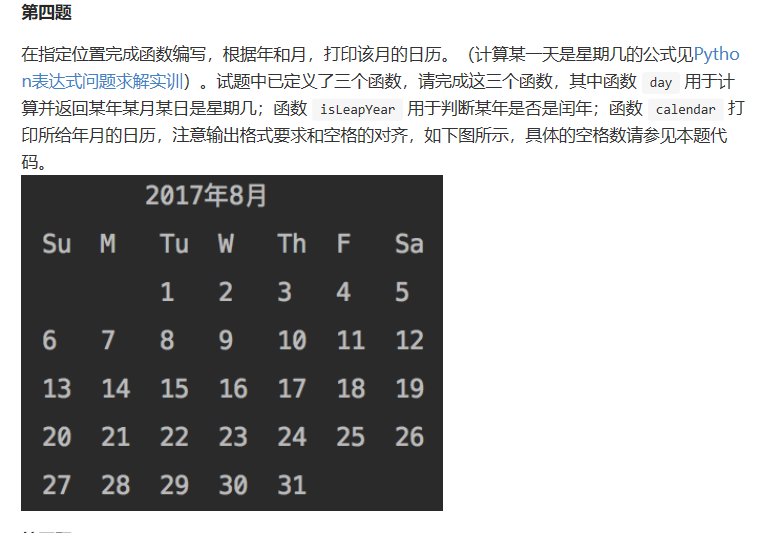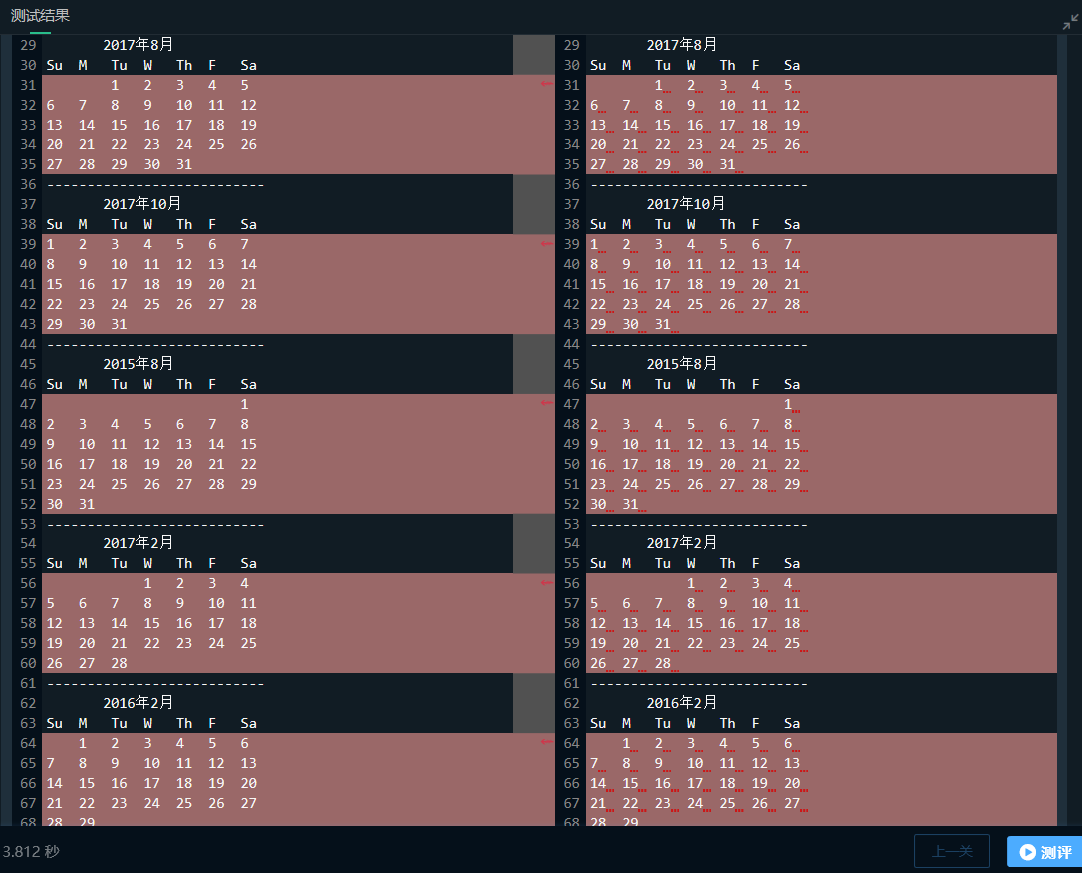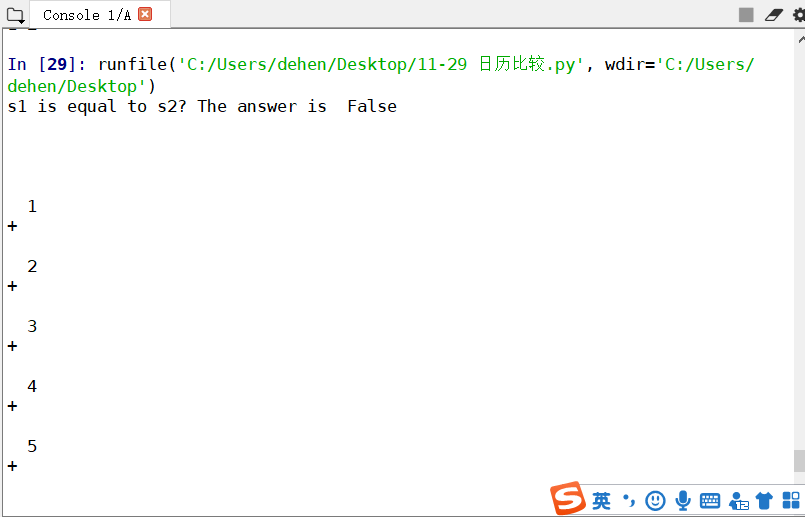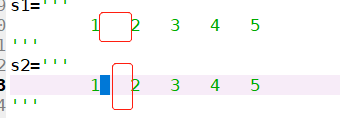# python中print函数的输出问题（空格，制表符）

## 问题描述educoder中判断程序是否正确，是通过输出结果的字符串匹配来判断的。

def day(y, m, d):#计算y年m月d日是星期几
# 请在下面编写代码
y0=y-(14-m)//12
x=y0+y0//4-y0//100+y0//400
m0=m+12*((14-m)//12)-2
d0=(d+x+(31*m0)//12)%7

# 请不要修改下面的代码
return d0

def isLeapYear(year): #判断year年是否闰年
# 请在下面编写代码
a=year%4
b=year%100
if(a==0 and b!=0):
isLeapYear="True"
else:
isLeapYear="False"
# 请不要修改下面的代码
return isLeapYear

def calendar(y, m): #打印y年m月日历
print('       {}年{}月'.format(y,m))
print('Su\tM\tTu\tW\tTh\tF\tSa')
# 请在下面编写代码
if(isLeapYear(y) is "True"):
list_1=[31,29,31,30,31,30,31,31,30,31,30,31]
firstday=day(y,m,1)
count=0
while(count<firstday):
print("\t",end="")
count+=1
a=1
while(a<=list_1[m-1]):
print(a,'\t',end="")
a+=1
count+=1
if(count%7==0):
print("")
if(a>list_1[m-1]):
print("")

else:
list_2=[31,28,31,30,31,30,31,31,30,31,30,31]
firstday=day(y,m,1)
count=0
while(count<firstday):
print("\t",end="")
count+=1
a=1
while(a<=list_2[m-1]):
print(a,'\t',end="")
a+=1
count+=1

if(count%7==0):
print("")
if(a>list_2[m-1]):
print("")## 解决方法

### 1 发现问题

import difflib
s1='''
1	2	3	4	5
'''
s2='''
1 	2 	3 	4 	5
'''

print('s1 is equal to s2 ? The answer is ',s1==s2)### 2 解决问题print(a,'\t',end="")


print('%d\t'%a,end="")


a=1
b=2
print(a,b)


### 3 程序通过## 总结

09-0321万+08-081240
01-201万+
05-141258
10-242万+
07-291071
01-284379
07-061万+
06-211万+
09-051万+
07-2211万+
07-312万+
08-1210万+
05-031万+
01-081万+
08-071万+